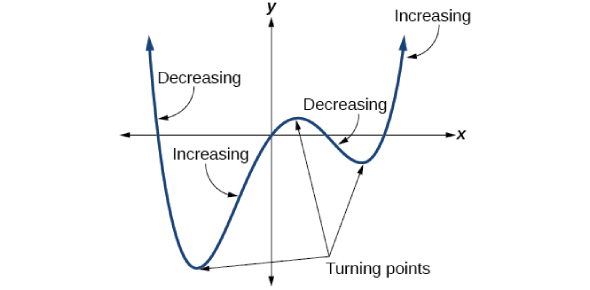# Features Of Polynomials

10 Questions | Total Attempts: 123Settings. What do the features of f' and f'' tell you about the original funciton f?

• 1.
For a polynomial P(x) defined on an interval [a,b], where do you need to check for minima and maxima?
• A.

Wherever P(x) = 0

• B.

Wherever P'(x) = 0

• C.

Wherever P''(x) = 0

• D.

At x = a and x = b

• 2.
Statement: An absolute (global) maximum is also a relative (local) maximum.
• A.

True

• B.

False

• 3.
Statement: Relative (local) extremes cannot occur at endpoints unless the slope of the tangent line at the endpoint is zero.
• A.

True

• B.

False

• 4.
Statement:  A point of inflection is found at any location where the second derivative of a function is zero.
• A.

True

• B.

False

• 5.
Statement:  At a location where the first derivative of a function is zero, the function may have a local minimum, a local maximum, or a point of inflection.
• A.

True

• B.

False

• 6.
Statement:  The slope of the tangent line at a point of inflection is always zero.
• A.

True

• B.

False

• 7.
Consider the polynomial f(x) and the sign line shown for its derivative f'(x). Which statement best describes the relative extrema of f?
• A.

The function f has relative minima at –2 and 5/2, and a relative maximum at 0

• B.

The function f has a relative minimum at 0 and a relative maximum at 5/2

• C.

The function f only has one relative extreme: a maximum at –2

• D.

The function f has a relative maximum at 0 and a relative minimm at 5/2

• 8.
Consider the polynomial f(x) and the sign line shown for its derivative f'(x). Which statement about increasing vs. decreasing is true?
• A.

The function f is increasing on the interval [–1,1]

• B.

The function f is decreasing on the inverval [–1,1]

• C.

The function f is decreasing on the interval [0,5/2]

• D.

The function f is overall an increasing function

• 9.
Consider the polynomial f(x) and the sign line shown for its derivative f'(x). Which statement about inflection points of f is definitely true?
• A.

The function f does not have any inflection points

• B.

The function f has an inflection point at –2 and possible inflection points at 0 and/or 5/2

• C.

The function f has an inflection point at –2 and potentially other inflection points, but NOT at 0 or 5/2

• D.

The function f has exactly four inflection points

Related Topics